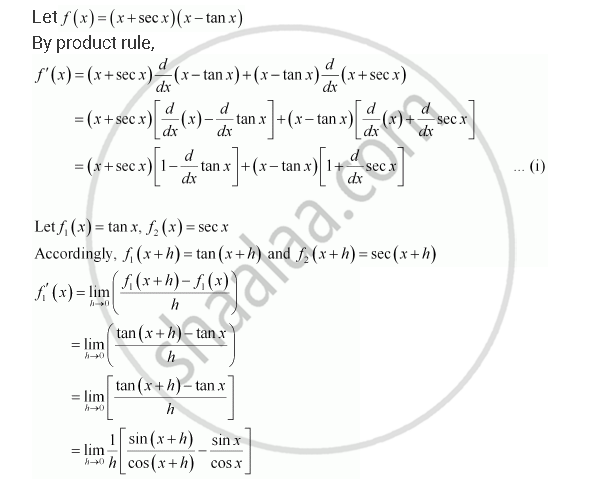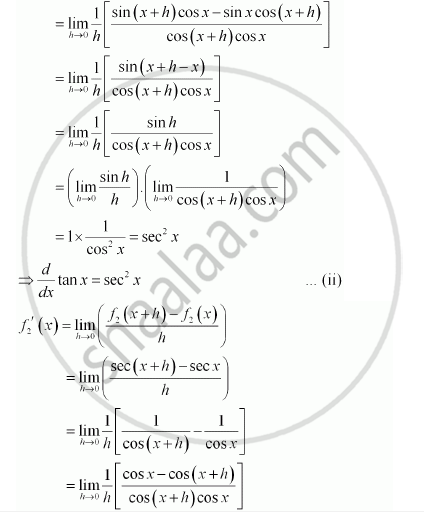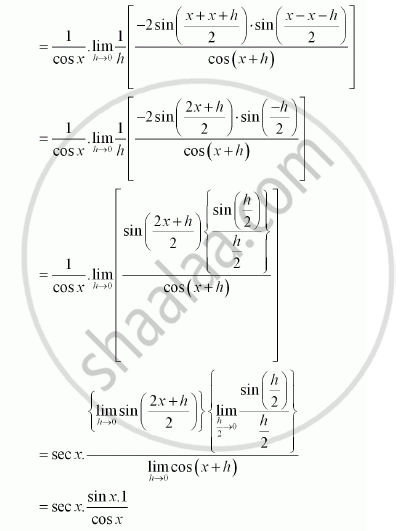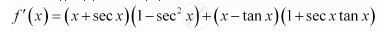CBSE (Commerce) Class 11CBSE
Share
Notifications

View all notifications
Books Shortlist
Your shortlist is empty

# Solution for Find the Derivative of the Following Functions (It is to Be Understood that A, B, C, D, P, Q, R and S Are Fixed Non-zero Constants and M and N Are Integers): (X + Sec X) (X – Tan X) - CBSE (Commerce) Class 11 - Mathematics

Login
Create free account

Forgot password?
ConceptDerivative Derivative of Polynomials and Trigonometric Functions

#### Question

Find the derivative of the following functions (it is to be understood that abcdp, q, r and s are fixed non-zero constants and m and n are integers): (x + sec x) (x – tan x)

#### Solution=> d/dx sec x = sec x tan x ...(iii)

From (i), (ii), and (iii), we obtainIs there an error in this question or solution?

#### APPEARS IN

NCERT Solution for Mathematics Textbook for Class 11 (2013 to Current)
Chapter 13: Limits and Derivatives
Q: 29 | Page no. 318
Solution Find the Derivative of the Following Functions (It is to Be Understood that A, B, C, D, P, Q, R and S Are Fixed Non-zero Constants and M and N Are Integers): (X + Sec X) (X – Tan X) Concept: Derivative - Derivative of Polynomials and Trigonometric Functions.
S